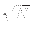Next: Theorem 1 Up: Grover's Algorithm Previous: Proof that Operations are   Contents

## Proof that Algorithm Increases Amplitude of Desired State

Having established that the transformations in question are unitary, and thus physically realizable, it is left to establish that iterations of Grover's algorithm increase the amplitude of the marked state C(Sm) = 1 enough that the probability of measuring state Sm is at least 1/2 in O() operations.

We start by examining the effect of the inversion about average operator A.

SubsectionsNext: Theorem 1 Up: Grover's Algorithm Previous: Proof that Operations are   Contents
Matthew Hayward - Quantum Computing and Grover's Algorithm GitHub Repository